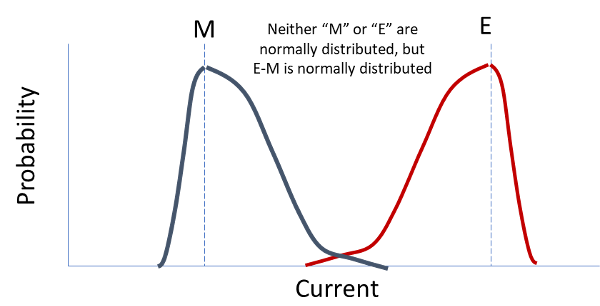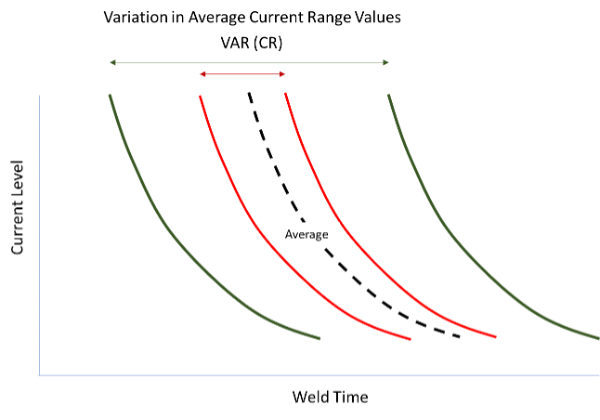top of page
Search

# Variation in Weld Data Concerning Expulsion and Minimum Weld Size and Current Level Conditions

Updated: Mar 30

#### Introduction:

To get predictable results from the resistance spot welding process it requires a correct theory of how the process operates and a correct expectation of how welding parameters interact to form a weld. Knowledgeable people often disagree about how the process works. For instance, an abstract question arose in an AWS D8D committee meeting about the nature of the variations in the expulsion condition, minimum weld size, and current level conditions. The statement was made that the current levels for these limits were “statistical” in nature. While these limits can be described by statistical procedures, it is important to note the underlying practical implications of how the weld grows and how these typical limits vary.

#### Discussion:

The origin of the inherent variations surrounding the minimum and expulsion conditions are best described by what these values represent. The minimum weld size condition is described by the act of weld nugget creation. The weld current that achieves this weld size is defined after a number of other welding conditions have been specified (electrode face diameter, weld force, weld time, etc.). The minimum weld size has a generally accepted definition (usually defined by 4 x √t, where t is the thickness in millimeters of the thinnest sheet). The action of specifying a minimum size will inherently skew the distribution of currents that are used to repeatedly measure this phenomenon as shown in Figure 1. The Minimum Current is skewed to the right of point “M”. It is more likely to measure minimum weld sizes at higher current levels vs “M”, but less likely to get minimum sizes at currents much below “M”. The practical implication of this in Design-of-Experiments (DoE) testing will be described later.

#### Figure 1 – Fundamental Relationships in the Variations in Minimum and Expulsion Currents due to Scatter from Repeated TestingSimilarly, the expulsion weld size condition is described by the act of weld nugget retention. The weld nugget has already been established (minimum condition) and continued increases in current produce increasing weld size. There are many papers describing this process. In any case, expulsion occurs when the weld size (diameter) grows in excess of the pinching forces impressed on the sheet surfaces by the electrode face and weld force that prohibit ejection of metal. Expulsion is avoided if the material at the weld periphery can effectively pinch the metal at edges of the molten pool (under hydrostatic pressure). The severity of expulsion is relatively complex, but includes the level of hydrostatic pressure, the cleanliness of the weld interface (faying interface), the ability to re-establish the seal surrounding the molten pool, etc. The Expulsion Current is skewed to the left of the Expulsion point, “E” in Figure 1. It is more likely to get expulsion to occur at lower current levels vs “E”, but less likely to extend the expulsion point to currents much above “E” on repeated testing. The practical implication of this in Design-of-Experiments (DoE) testing will be described later. There are fundamental differences between the nature of minimum and expulsion points in current range testing. The minimum current level is a very unstable welding condition (vs “E”). It is affected by all of the common weld factors (electrode face diameter, weld force, weld time, material conditions, etc.) and all of the” two-factor” interactions between welding parameters. Nearly any change in the welding conditions can easily alter the minimum weld size. Consequently, it becomes a “snap-shot” of welding conditions at that instance. Conversely, expulsion is a relatively stable welding condition (vs “M”). It is generally affected only by selections of electrode face diameter, weld time, weld force, and a “two-factor” interaction between weld time and weld force. The list of conditions influencing expulsion are relatively “established” by their nature. This is not to indicate that there is not variation in the current levels that establish these conditions, but rather to describe the relative stability of the two phenomena. The current range (CR) is a normally distributed quantity because it represents the difference in current levels between two conditions. Since it is typically normally distributed, it is responsive to the variations in the minimum and expulsion weld current levels. There are some well established relationships (mathematical equations) that can relate the variations in these quantities. But first, the concept of COVARIANCE is needed. Covariance is always described by the relative relationship between two quantities. It is the ability to predict the value of one quantity when the other quantity is known. In other words, if one is doing repeated current range testing (same materials, same parameters), then if the minimum current is known, then it is possible to “guess” the value of the expulsion current based on past results. Or, in defining the limits on a weld lobe, once we know the minimum current and we have established the first expulsion point, we can make a good “guess” on the next expulsion point (assuming the electrode face had not substantially changed in the process). We inherently use this relationship, without realizing it, when we perform current range testing. There is a high COVARIANCE between “M” and “E” (e.g. COV(M,E)). Or, when either “E” or “M” is known, one can generally predict the occurrence of the other condition (“guess” the current where it will occur). The mathematical relationship is:

VAR(E-M) = VAR (E) + VAR(M) – 2COV(M,E)

Where VAR is the variance (square of the standard deviation) and COV is defined in many textbooks. For our purposes, VAR and COV represent variability in test data. The equation above can be re-arranged into:

VAR (E) + VAR(M) = VAR(E-M) + 2COV(M,E)

(Random current variations) = (Variation in CR) + (Covariance M,E)

This relationship says that the sum of the variations in current range testing can be broken down into variations in current range (CR) widths and variations in current levels (minimum and expulsion). This is shown as current level and current range variations in Figures 2 and 3. Figure 2 shows the variation in current ranges. The green and the red current ranges vary in width about an average line. This is referenced by the horizontal bars above these two current ranges. Figure 3 shows the variation in current levels. The tan (“A”), gray (“B”), and the red (“C”) current ranges have the same current range values but vary in current level about an average line. The current ranges shift to lower or higher current levels. The shifts in current levels in Figure 3 (horizontal lines for A, B, and C) can be referenced relative to the same horizontal lines as used in Figure 2 to describe variations in current range (horizontal lines above the curves).

#### Figure 2 - Fundamental Relationship in Variation in Current Range due to Scatter from Repeated Testing#### Figure 3 - Fundamental Relationship in Variation in Current Level due to Scatter from Repeated TestingThe practical implications of these findings have many applications for improving our understanding of current range test data. One of the most important applications is its use in Design-of-Experiments (DoE) testing. Because of the skewed nature of the minimum and expulsion current lines, a correct interpretation of current range testing is obtained by using minimum weld size and expulsion conditions as inputs to the DoE, rather than using the current levels themselves. The DoE analysis is built on the assumptions that the data is normally distributed. Fundamentally, it is not reasonable to expect the current levels to be permitted to vary towards infinity, which is one of the assumptions of normally distributed data. Used properly, the currents level data are represented as measurements, rather than inputs, in the DoE. Consequently, an appropriate transformation of the current levels will be required to correctly estimate these values. If the current levels are substituted in place of the phenomena they represent, then the weld robustness data will become skewed since the levels in the DoE are internal and perhaps external replicated many times in a DoE. The important input factors are built from how well variations in contrasting data are accounted within the DoE. Literally hundreds of DoE experiments have been planned, executed, and analyzed using this approach by the author. This fundamental shift in the approach to DoE testing improves the predictions of current levels, current ranges, and our understanding (interpretation) of the test results.

The author, Warren Peterson has performed hundreds of DoEs on nearly all forms of welding processes. This and many on-site problem-solving activities have provided a broad and deep understanding of the various welding processes. In this case, Warren has discussed the phenomena surrounding resistance welding process optimizations. This work can be done at customer facilities to achieve specific customer-orientated goals. In most cases, United Technical can find a practical solution to your challenge. Please contact us to discuss how we can help you.